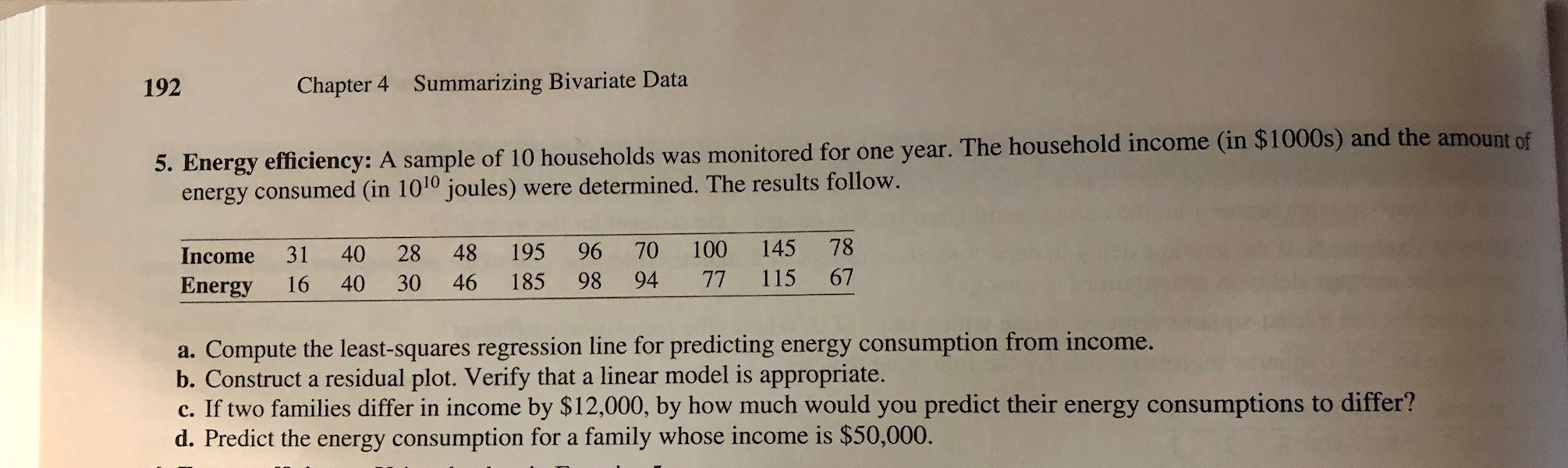192Chapter 4Summarizing Bivariate Data5. Energy efficiency: A sample of 10 households was monitored for one year. The household income (in \$1000s) and the amount ofenergy consumed (in 1010 joules) were determined. The results follow.Income 31 40 28 48 195 96 70 100 145 78Energy16 40 30 46 185 98 94 77 115 67a. Compute the least-squares regression line for predicting energy consumption from income.b. Construct a residual plot. Verify that a linear model is appropriate.c. If two families differ in income by \$12,000, by how much would you predict their energy consumptions to differ?d. Predict the energy consumption for a family whose income is \$50,000.

Question

How would I do part a?

How do I construct a residual plot for part b?

And how would I do part D?help_outlineImage Transcriptionclose192 Chapter 4 Summarizing Bivariate Data 5. Energy effici ency: A sample of 10 households was monitored for one year. The household income (in \$1000s) and the amount of energy consumed (in 1010 joules) were determined. The results follow. Income 31 40 28 48 195 96 70 100 145 78 Energy 16 40 30 46 185 98 94 77 115 67 a. Compute the least-squares regression line for predicting energy consumption from income. b. Construct a residual plot. Verify that a linear model is appropriate. c. If two families differ in income by \$12,000, by how much would you predict their energy consumptions to differ? d. Predict the energy consumption for a family whose income is \$50,000. fullscreen
Step 1

Given data of a sample of 10 households for one year. The household income in \$1000s and the amount of energy consumed in (1010 joules) as shown below

The reg...

Want to see the full answer?

See Solution

Want to see this answer and more?

Our solutions are written by experts, many with advanced degrees, and available 24/7

See Solution
Tagged in

Advanced Topics in Statistics